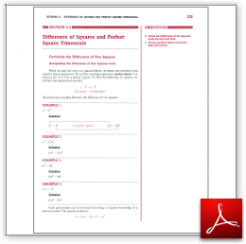Algebra Math Book - Introductory Algebra Thank you for your continual support.

 The power of mathematics rests in the logic of thinking.ID: Sec5-2
Description: Factoring the Difference of Two Squares-Perfect Square Trinomial
Price: 1.99

# Algebra Math book - Introductory Algebra - Chapter 5 - Section 2 - Difference of Squares and Perfect Square Trinomials

## Section 5.2 - Objectives

2.  Given the difference ot two squares, write the factored form.

3.  Given a perfect square trinomial, state the factors.

Navigate to
Previous Section or Next Section
or Chapter 5 Details or Other Chapters

This section of my algebra math book, Introductory Algebra, also includes in the download:

• Cover Sheet